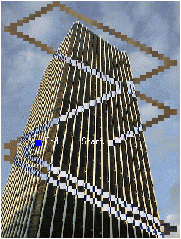## Animation

The animation of graphics on a screen reuses techniques of animated drawings. The major part of a drawing does not change, only the animated part must modify the color of its constituent pixels. One of the immediate problems we meet is the speed of animation. It can vary depending on the computational complexity and on the execution speed of the processor. Therefore, to be portable, an application containing animated graphics must take into account the speed of the processor. To get smooth rendering, it is advisable to display the animated object at the new position, followed by the erasure of the old one and taking special care with the intersection of the old and new regions.

##### Moving an object
We simplify the problem of moving an object by choosing objects of a simple shape, namely rectangles. The remaining difficulty is knowing how to redisplay the background of the screen once the object has been moved.

We try to make a rectangle move around in a closed space. The object moves at a certain speed in directions X and Y. When it encounters a border of the graphical window, it bounces back depending on the angle of impact. We assume a situation without overlapping of the new and old positions of the object. The function calc_pv computes the new position and the new velocity from an old position (x`,`y), the size of the object (sx`,`sy) and from the old speed (dx`,`dy), taking into account the borders of the window.
```# let` `calc_pv` `(x`,`y)` `(sx`,`sy)` `(dx`,`dy)` ``=`` `` `` `` `let` `nx1` ``=`` `x`+`dx` `` `` `` `` `and` `` `ny1` ``=`` `y` ``+`` `dy` `` `` `and` `nx2` ``=`` `x`+`sx`+`dx` `` `and` `` `ny2` ``=`` `y`+`sy`+`dy` `` `` `and` `ndx` ``=`` `ref` `dx` `` `` `and` `` `ndy` ``=`` `ref` `dy` `` `` `` `in` `` `` `` `` `` `` `(` `if` `(nx1` ``<`` ``0`)` ``||`` `(nx2` ``>=`` `Graphics.size_x())` `then` `ndx` ``:=`` ``-`dx` `);` `` `` `` `` `(` `if` `(ny1` ``<`` ``0`)` ``||`` `(ny2` ``>=`` `Graphics.size_y())` `then` `ndy` ``:=`` ``-`dy` `);` `` `` `` `` `((x`+`` ``!`ndx`,`` `y`+`` ``!`ndy)`,`` `(`!`ndx`,`` ``!`ndy));;`val calc_pv :``  int * int -> int * int -> int * int -> (int * int) * (int * int) = <fun>`

```
The function move_rect moves the rectangle given by pos and size n times, the trajectory being indicated by its speed and by taking into account the borders of the space. The trace of movement which one can see in figure 5.7 is obtained by inversion of the corresponding bitmap of the displaced rectangle.
```# let` `move_rect` `pos` `size` `speed` `n` ``=`` `` `` `let` `(x`,`` `y)` ``=`` `pos` `and` `(sx`,`sy)` ``=`` `size` `in` `` `` `let` `mem` ``=`` `ref` `(Graphics.get_image` `x` `y` `sx` `sy)` `in` `` `` `` `let` `rec` `move_aux` `x` `y` `speed` `n` ``=`` `` `` `` `if` `n` ``=`` ``0`` `then` `Graphics.moveto` `x` `y` `` `` `` `else` `` `` `` `` `` `let` `((nx`,`ny)`,`n_speed)` ``=`` `calc_pv` `(x`,`y)` `(sx`,`sy)` `speed` `` `` `` `` `` `and` `old_mem` ``=`` ``!`mem` `in` `` `` `` `` `` `` `mem` ``:=`` `Graphics.get_image` `nx` `ny` `sx` `sy;` `` `` `` `` `` `Graphics.set_color` `Graphics.blue;` `` `` `` `` `` `Graphics.fill_rect` `nx` `ny` `sx` `sy;` `` `` `` `` `` `Graphics.draw_image` `(inv_image` `old_mem)` `x` `y;` `` `` `` `` `` `move_aux` `nx` `ny` `n_speed` `(n`-``1`)` `` `` `in` `move_aux` `x` `y` `speed` `n;;`val move_rect : int * int -> int * int -> int * int -> int -> unit = <fun>`

```

The following code corresponds to the drawings in figure 5.7. The first is obtained on a uniformly red background, the second by moving the rectangle across the image of Jussieu.

```# let` `anim_rect` `()` ``=`` `` `` `` `Graphics.moveto` ``1``0``5`` ``1``2``0`;` `` `` `Graphics.set_color` `Graphics.white;` `` `` `Graphics.draw_string` ``"Start"`;` `` `` `move_rect` `(`1``4``0``,``1``2``0`)` `(`8``,``8`)` `(`8``,``4`)` ``1``5``0`;` `` `` `let` `(x`,`y)` ``=`` `Graphics.current_point()` `in` `` `` `` `` `Graphics.moveto` `(x`+``1``3`)` `y;` `` `` `Graphics.set_color` `Graphics.white;` `` `` `Graphics.draw_string` ``"End"`;;`val anim_rect : unit -> unit = <fun>`# anim_rect();;`- : unit = ()`

```Figure 5.7: Moving an object.

The problem was simplified, because there was no intersection between two successive positions of the moved object. If this is not the case, it is necessary to write a function that computes this intersection, which can be more or less complicated depending on the form of the object. In the case of a square, the intersection of two squares yields a rectangle. This intersection has to be removed.